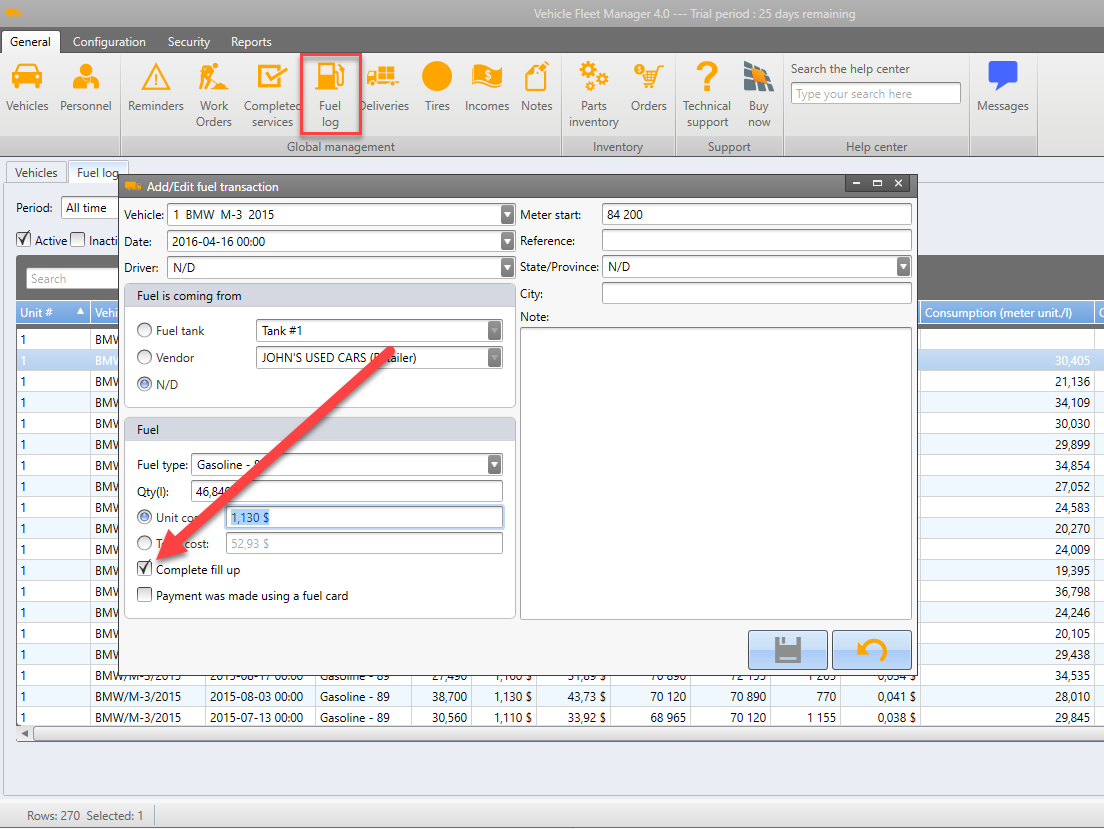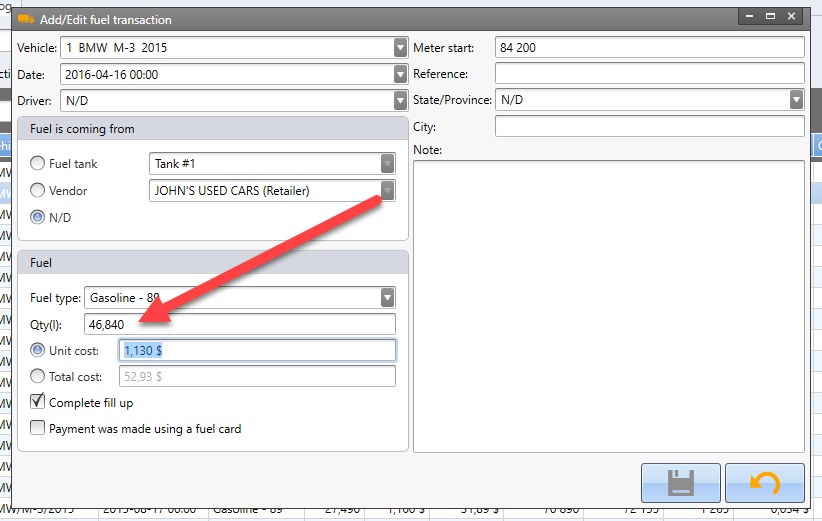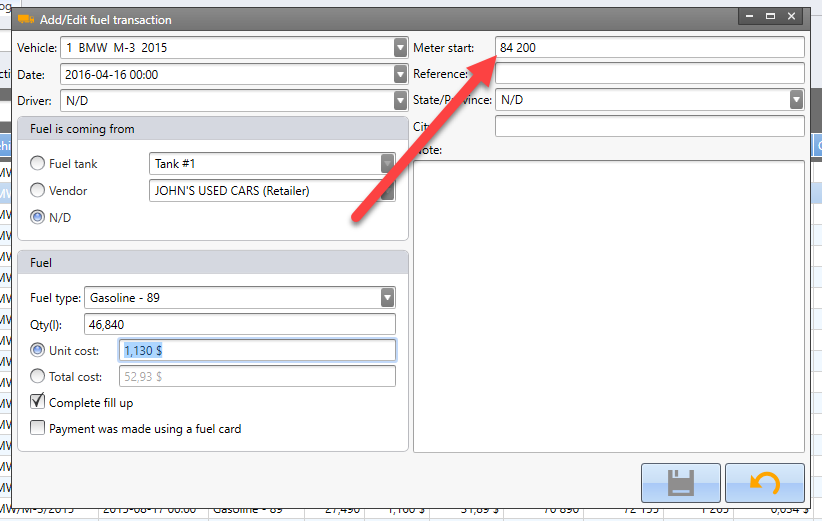# How fuel consumption is calculated?

Vincent Trepanier -

The first thing to know about fuel consumption calculation is that it is mathematically possible to calculate consumption only if a complete fill up is performed at the tank. So when adding a new fuel transaction in the software, you have to check this box to tell it that a complete fill up has been performed :You also have to enter the quantity of fuel you put into the vehicle :Then finally, you have to enter a "Meter start" value. It's the odometer value at the time of fueling the vehicle :With those three values, the software is able to calculate fuel consumption accurately. You dont need to specify a "Meter end" value as the software assumes the "Meter end" of a fuel record is the "Meter start" of the following fuel record.

Now there is two columns displaying the consumption, it's two different standard calculation :
1. Consumption (meter unit/vol unit) :
For example, 16 miles/Gallon

2. Consumption (Vol unit/100 meter unit) :
For example, 9 Liters/100 Km